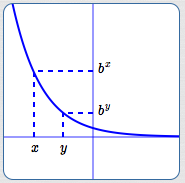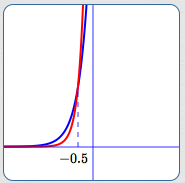﻿ Exponential Functions: Review and Additional Properties

# EXPONENTIAL FUNCTIONS: REVIEW AND ADDITIONAL PROPERTIES

• PRACTICE (online exercises and printable worksheets)

An exponential function is a function of the form $\,f(x) = b^x\,$ for $\,b > 0\,$ and $\,b\ne 1\,$.
Notice that the variable is in the exponent.
For example, $\,y = 2^x\,$, $\,y = (\frac 12)^x\,$, and $\,y = \text{e}^x\,$ are exponential functions.

Exponential functions and their graphs were introduced in the Algebra II curriculum:

Additional higher-level information that is important for Precalculus is presented below.

## Increasing/Decreasing Properties of Exponential Functions

 When $\,b > 1\,$, $\,f(x) = b^x\,$ is an increasing function. That is, for all real numbers $\,x\,$ and $\,y\,$: $$x < y \ \ \Rightarrow\ \ b^x < b^y$$ It is clear from the graph that the other direction is also true: $$b^x < b^y \ \ \Rightarrow\ \ x < y$$ Together, we have: $$x < y\ \ \text{is equivalent to}\ \ b^x < b^y \tag{1}$$ Notice that when the base is greater than one, the inequality symbols that compare the inputs ($\,x\,$ and $\,y\,$) and their corresponding outputs ($\,b^x\,$ and $\,b^y\,$) have the same direction: $$x \ {\bf\color{red}{\lt }}\ y\ \ \text{is equivalent to}\ \ b^x\ {\bf\color{red}{\lt }}\ b^y \tag{1a}$$ This equivalence can be alternately stated as: $$x \ {\bf\color{red}{>}}\ y\ \ \text{is equivalent to}\ \ b^x\ {\bf\color{red}{>}}\ b^y \tag{1b}$$$y = b^x\,$, for $\,b > 1\,$ an increasing exponential function $x < y \ \ \iff\ \ b^x < b^y$ When $\,0 < b < 1\,$, $\,f(x) = b^x\,$ is a decreasing function. That is, for all real numbers $\,x\,$ and $\,y\,$: $$x < y \ \ \Rightarrow\ \ b^x > b^y$$ It is clear from the graph that the other direction is also true: $$b^x > b^y \ \ \Rightarrow\ \ x < y$$ Together, we have: $$x < y\ \ \text{is equivalent to}\ \ b^x > b^y \tag{2}$$ Notice that when the base is between zero and one, the inequality symbols that compare the inputs ($\,x\,$ and $\,y\,$) and their corresponding outputs ($\,b^x\,$ and $\,b^y\,$) have different directions: $$x \ {\bf\color{red}{<}}\ y\ \ \text{is equivalent to}\ \ b^x\ {\bf\color{red}{>}}\ b^y \tag{2a}$$ This equivalence can be alternately stated as: $$x \ {\bf\color{red}{>}}\ y\ \ \text{is equivalent to}\ \ b^x\ {\bf\color{red}{<}}\ b^y \tag{2b}$$$y = b^x\,$, for $\,0 < b < 1\,$ a decreasing exponential function $x < y \ \ \iff\ \ b^x > b^y$

## Exponential Functions are One-to-One

The graphs of exponential functions pass both vertical and horizontal lines tests, so they are one-to-one functions.
Thus: $$x = y \ \ \text{is equivalent to}\ \ b^x = b^y \tag{3}$$ Consequently, exponential functions have inverses.
In a future section, we'll see that the class of logarithmic functions provide inverses to the class of exponential functions.

## Solving Inequalities involving Exponential Functions

Equivalences (1), (2), and (3) can be used to easily solve certain types of mathematical sentences, as illustrated in the following examples.

 Solve: $2^{3x-1} < 2^{5x}$ This is an inequality of the form $$\,b^{\text{stuff1}} < b^{\text{stuff2}}\,$$ where the base, $\,b\,$, is $\,2\,$. Notice that the exponential functions on both sides use the same base. In this example, the base is greater than one, so we'll use equivalence (1): $$x < y \ \ \iff\ \ b^x < b^y$$ SOLUTION: \begin{alignat}{2} 2^{3x-1} \ &<\ 2^{5x} \qquad&&\text{original inequality}\cr\cr 3x-1 \ &<\ 5x&&\text{use (1); inequality symbol doesn't change}\cr\cr -2x\ \ &<\ 1&&\text{addition property of inequality}\cr\cr x\ \ &>\ -\frac12&&\text{divide by a negative #; reverse inequality} \end{alignat}blue curve:   $y = 2^{3x - 1}$ red curve:   $y = 2^{5x}$ blue curve lies below red curve for $\,x > -\frac 12$ Solve: $\displaystyle\frac 1{3^{x^2-1}} > 1$ This inequality will be solved in two different ways. As long as you use correct tools in a correct way, there are often different ways you can proceed. SOLUTION #1: \begin{alignat}{2} \frac 1{3^{x^2-1}} \ &>\ 1 \qquad&&\text{original inequality}\cr\cr \left(\frac 13\right)^{x^2-1}\ &>\ \left(\frac 13\right)^0 \ \ &&\text{rename both sides using exponent laws}\cr\cr x^2 - 1\ \ &<\ 0&&\text{use (2); inequality changes direction}\cr\cr x^2\ \ &<\ 1&&\text{addition property of inequality}\cr\cr -1 < \ &x\ < 1&&\text{inspection (knowledge of x^2 curve)} \end{alignat} SOLUTION #2: \begin{alignat}{2} \frac 1{3^{x^2-1}} \ &>\ 1 \qquad&&\text{original inequality}\cr\cr 1\ &>\ 3^{x^2-1} \ \ \ \ &&\text{multiply both sides by 3^{x^2-1}\overset{\text{always}}{>}0}\cr\cr 3^0 \ &>\ 3^{x^2-1}&&\text{rename: 1 = 3^0}\cr\cr 0\ \ &>\ x^2 - 1\ \ &&\text{use (1)}\cr\cr x^2 \ &<\ 1&&\text{addition property; re-arrange}\cr\cr -1 < \ &x\ < 1&&\text{inspection (knowledge of x^2 curve)} \end{alignat}blue curve:   $\displaystyle y = \frac{1}{3^{x^2-1}}$ red curve:   $y = 1$ blue curve lies above red curve for $\,x\,$ between $\,-1\,$ and $\,1\,$
Master the ideas from this section